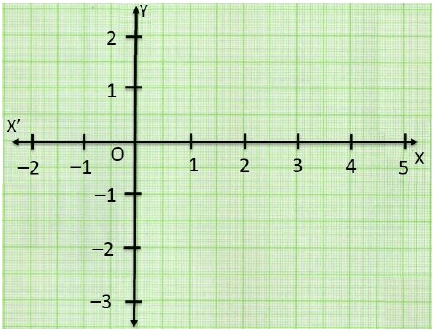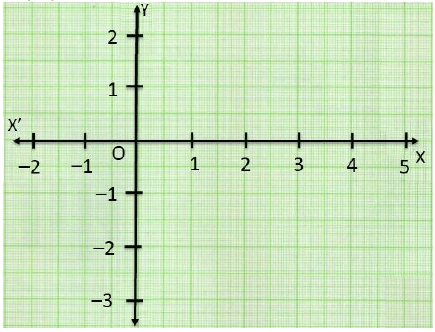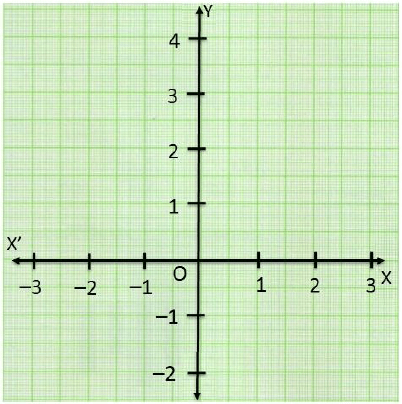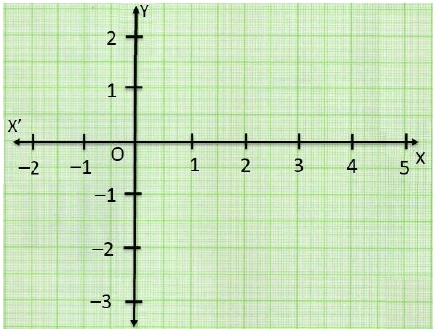# Test: Coordinate Geometry- Assertion & Reason Type Questions

## 10 Questions MCQ Test Mathematics (Maths) Class 9 | Test: Coordinate Geometry- Assertion & Reason Type Questions

Description
Attempt Test: Coordinate Geometry- Assertion & Reason Type Questions | 10 questions in 20 minutes | Mock test for Class 9 preparation | Free important questions MCQ to study Mathematics (Maths) Class 9 for Class 9 Exam | Download free PDF with solutions
QUESTION: 1

### DIRECTION : In the following questions, a statement of assertion (A) is followed by a statement of reason (R). Mark the correct choice as:Assertion : The point (0, 4) lies on y -axis.Reason : The x coordinate on the point on y -axis is zero.

Solution:

We know that the if the point lies on y-axis, its x-coordinate is 0.

So, Reason is correct.

The x coordinate of the point (0, 4) is zero.

So, Point (0, 4) lies on y -axis.

So, Assertion is also correct Correct option is (a) Both assertion (A) and reason (R) are true and reason (R) is the correct explanation of assertion (A).

QUESTION: 2

### DIRECTION : In the following questions, a statement of assertion (A) is followed by a statement of reason (R). Mark the correct choice as:Assertion: Point (4, -2) lies in IV quadrant.Reason: The perpendicular distance of a point from the y-axis is called its abscissa.

Solution:We know that the perpendicular distance of a point from y-axis is called its x-coordinate or abscissa.

So, Reason is correct.

Point (4, -2) lies in IV quadrant.

So, Assertion is also correct but Reason is not the correct explanation of Assertion. Correct option is (b) Both assertion (A) and reason (R) are true and reason (R) is not the correct explanation of assertion (A).

QUESTION: 3

### DIRECTION : In the following questions, a statement of assertion (A) is followed by a statement of reason (R). Mark the correct choice as:Assertion : If the ordinate of a point is equal to its abscissa, then the point lies either in the first quadrant or in the second quadrant.Reason : A point both of whose coordinates are negative will lie in third quadrants.

Solution: We know that A point both of whose coordinates are negative will lie in third quadrants. So, Reason is correct.

Also we know that If the ordinate of a point is equal to its abscissa, then the point lies either in the first quadrant or in the third quadrant. So, Assertion is not correct

Correct option is (d) Assertion (A) is false but reason (R) is true.

QUESTION: 4

DIRECTION : In the following questions, a statement of assertion (A) is followed by a statement of reason (R). Mark the correct choice as:

Assertion: Point A(-2, -4) lies on III quadrant

Reason: A point both of whose coordinates are negative lies in III quadrant

Solution: We know that a point both of whose coordinates are negative lies in III quadrant

So, Reason is correct.

Hence, Point A(-2, -4) lies on III quadrant So, Assertion is also correct and Reason explains Assertion

Correct option is (a) Both assertion (A) and reason (R) are true and reason (R) is the correct explanation of assertion (A).

QUESTION: 5

DIRECTION : In the following questions, a statement of assertion (A) is followed by a statement of reason (R). Mark the correct choice as:

Assertion: A point whose abscissa is 2 and ordinate is -3 lies in fourth quadrant

Reason: Points of the type (–, +) lie in the second quadrant.

Solution:

We know that Points of the type (–, +) lie in the second quadrant. So, Reason is correct.

Also, we know that Points of the type (+, –) lie in the fourth quadrant. Hence, point whose abscissa is 2 and ordinate is -3 lies in fourth quadrant So, Assertion is also correct but Reason is the not the correct explanation of Assertion.

Correct option is (b) Both assertion (A) and reason (R) are true but reason (R) is not the correct explanation of assertion (A).

QUESTION: 6

DIRECTION : In the following questions, a statement of assertion (A) is followed by a statement of reason (R). Mark the correct choice as:

Assertion: The abscissa of a point (5, 2) is 5.

Reason: The perpendicular distance of a point from y-axis is called its abscissa.

Solution:We know that the perpendicular distance of a point from y-axis is called its x-coordinate or abscissa.

So, Reason is correct.

The x co-ordinate of the point (5, 2) is 5.

So, Assertion is also correct

Correct option is (a) Both assertion (A) and reason (R) are true and reason (R) is the correct explanation of assertion (A).

QUESTION: 7

DIRECTION : In the following questions, a statement of assertion (A) is followed by a statement of reason (R). Mark the correct choice as:

Assertion : The point (-2, 0) lies on y -axis and (0, 4) on x -axis.

Reason : Every point on the x -axis has zero distance from x -axis and every point on the y -axis has zero distance from y -axis.

Solution:We know that Every point on the x -axis has zero distance from x -axis and every point on the y -axis has zero distance from y -axis. So, Reason is correct.

Now, point (-2, 0) lies on x-axis and (0, 4) on y-axis So, Assertion is not correct Correct option is (d) Assertion (A) is false but reason (R) is true.

QUESTION: 8

DIRECTION : In the following questions, a statement of assertion (A) is followed by a statement of reason (R). Mark the correct choice as:

Assertion : The points (-1, 2) and (2,- 1) are at different positions in the coordinate plane.

Reason : Point (-1, 2) lies in II-quadrant and (2,- 1) lies in IV quadrant

Solution:We know that Point (-1, 2) lies in II-quadrant and (2,- 1) lies in IV quadrant So, Reason is correct.

Hence the points (-1, 2) and (2,- 1) are at different positions in the coordinate plane. So, Assertion is also correct Correct option is (a) Both assertion (A) and reason (R) are true and reason (R) is the correct explanation of assertion (A).

QUESTION: 9

DIRECTION : In the following questions, a statement of assertion (A) is followed by a statement of reason (R). Mark the correct choice as:

Assertion: The perpendicular distance of the point A(3, 4) from the y-axis is 4

Reason: The perpendicular distance of a point from y-axis is called its x-coordinate.

Solution:

We know that the perpendicular distance of a point from y-axis is called its x-coordinate or abscissa.

So, Reason is correct.

The x co-ordinate of the point (3, 4) is 3.

So, Assertion is not correct Correct option is (d) Assertion (A) is false but reason (R) is true.

QUESTION: 10

DIRECTION : In the following questions, a statement of assertion (A) is followed by a statement of reason (R). Mark the correct choice as:

Assertion: A point whose abscissa is -3 and ordinate is 2 lies in second quadrant

Reason: Points of the type (–, +) lie in the second quadrant.

Solution:

We know that Points of the type (–, +) lie in the second quadrant. So, Reason is correct.

Hence, point whose abscissa is -3 and ordinate is 2 lies in second quadrant So, Assertion is also correct and Reason explains Assertion Correct option is (a) Both assertion (A) and reason (R) are true and reason (R) is the correct explanation of assertion (A).Use Code STAYHOME200 and get INR 200 additional OFF Use Coupon Code